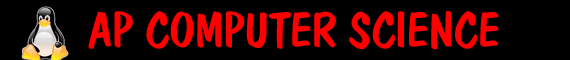Quiz 9- Control, If Statements       id: 1. True or false: Equality and relational operators (==,>,8) System.out.print("p"); else System.out.print("j"); System.out.print("f"); ```   p jf pj pf   3. What is printed by the following statement: ```int x=10; if (x>8) System.out.print("p"); if (x>12) System.out.print("j"); else System.out.print("f"); ``` p pj pjf pf